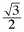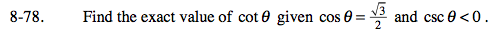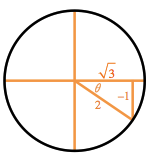### Home > PC > Chapter 8 > Lesson 8.2.1 > Problem8-78

8-78.

Find the exact value of cot θ given cos θ =and csc θ < 0. Homework Help ✎Since the csc(θ) < 0, it must be in Quadrants III or IV.
Since the cos(θ) > 0, it must be in Quadrant IV.

Sketch a diagram and label.

$\text{cot}(\theta)=\frac{\text{adjacent side}}{\text{opposite side}}$